Latest Banking jobs   »   Quantitative Aptitude Quiz For IBPS Clerk...

Quantitative Aptitude Quiz For IBPS Clerk Prelims 2021- 1st December

Directions (1-5): In the given questions, two quantities are given, one as ‘Quantity I’ and another as ‘Quantity II’. You must determine relationship between two quantities and choose the appropriate option: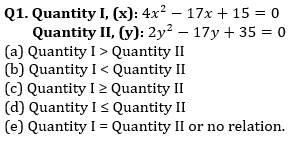Q2. Quantity I, (Time taken by A to complete the work alone): Time taken by A & B together to complete a piece of work is equal to time taken by C to complete the same work alone. B working alone can complete same piece of work in 45 days and C is 125% more efficient than B.
Quantity II: 27 days.
(a) Quantity I > Quantity II
(b) Quantity I < Quantity II
(c) Quantity I ≥ Quantity II
(d) Quantity I ≤ Quantity II
(e) Quantity I = Quantity II or no relation.

Q3. Quantity I, (Rate of interest): A man invested equal amount of money for 2 years in two schemes – A & B. Scheme – A & B offers SI and CI (compounding annually) respectively. Both schemes offers same rate of interest. Ratio of simple interest received to compound interest received after 2 years is 10 : 11.
Quantity II: 16%
(a) Quantity I > Quantity II
(b) Quantity I < Quantity II
(c) Quantity I ≥ Quantity II
(d) Quantity I ≤ Quantity II
(e) Quantity I = Quantity II or no relation.

Q4. Train – A crosses a pole in 12.5 seconds. Ratio of length of train – A to that of B is 5 : 8 and speed of train – A is double of that of train – B. Length of train – B is 800m.
Quantity I: Time taken by train – A to cross train – B while running in same direction.
Quantity II: 65 seconds.
(a) Quantity I > Quantity II
(b) Quantity I < Quantity II
(c) Quantity I ≥ Quantity II
(d) Quantity I ≤ Quantity II
(e) Quantity I = Quantity II or no relation.

Q5. Quantity I, (Total surface area of 1 hemispherical bowl): A spherical ball of radius 42 cm is melted to form 16 identical hemispherical bowls.
Quantity II, (Curved surface area of a cylindrical pipe): Volume of each cylindrical pipe is 12320 cm3. Ratio of radius to that of height of cylindrical pipe is 7 : 10.
(a) Quantity I > Quantity II
(b) Quantity I < Quantity II
(c) Quantity I ≥ Quantity II
(d) Quantity I ≤ Quantity II
(e) Quantity I = Quantity II or no relation.

Directions (6-10): Each of the following questions below consists of a question and two statements numbered I and II given. You have to decide whether the data provided in the statements is sufficient to answer the questions.
(a) if the data given in statement I alone is sufficient to answer the question while the data in statement II alone is not sufficient to answer the question.
(b) if the data given in statement II alone is sufficient to answer the question while the data in statement I alone is not sufficient to answer the question.
(c) if the data either in statement I alone or in statement II alone is sufficient to answer the question.
(d) If the data in neither statement I nor II is sufficient to answer the question.
(e) If the data in both statements I and II together is necessary to answer the question.

Q6. Find the cost price of article by shopkeeper on selling the article at Rs. 240 ?
(I) If the article sold at 25% more the profit earned will be Rs. 40.
(II) Marked price of article is Rs. 400 and profit% is equal to discount% and profit% is 40%.

Q7. Find the volume of right circular cone ?
(I) Height of cone is 100% more than radius of cone.
(II) Area of base of cone is 154 cm².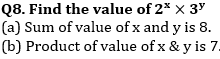Q9. Find the speed of boat in still water?
(I) Time taken by boat to cover 64 km in downstream is half the time taken by same boat to cover same distance in still water.
(II) Speed of stream is 5 km/hr

Q10. In a box three types of balls are there, Black, Red and White. If no. of white balls is given then find out the probability of getting one white ball.
(I) Probability of getting one Red ball is given.
(II) Probability of getting one black ball is given.

Directions (11-15): In the following questions, two quantities (I) and (II) are given. You have to solve both the quantity and mark the answer accordingly.(Compare only magnitude of Quantity I & Quantity II )

Q11. Quantity I, (Time taken by A, B & C together to compete the work): A & C working together can complete a piece of work in 6 days and B working alone can complete same piece of work in 18 days. A is 20% more efficient than B.
Quantity II: 3 days.
(a) Quantity I < Quantity II
(b) Quantity I > Quantity II
(c) Quantity I ≤ Quantity II
(d) Quantity I ≥ Quantity II
(e) Quantity I = Quantity II or no relation.

Q12. Quantity I, (Value of R): Deepak invested Rs.6000 each at R% p.a. SI and R% p.a. CI compounding annually respectively for 2 years. Total CI received is Rs.86.4 more total SI received.
Quantity II: 28
(a) Quantity I < Quantity II
(b) Quantity I > Quantity II
(c) Quantity I ≤ Quantity II
(d) Quantity I ≥ Quantity II
(e) Quantity I = Quantity II or no relation.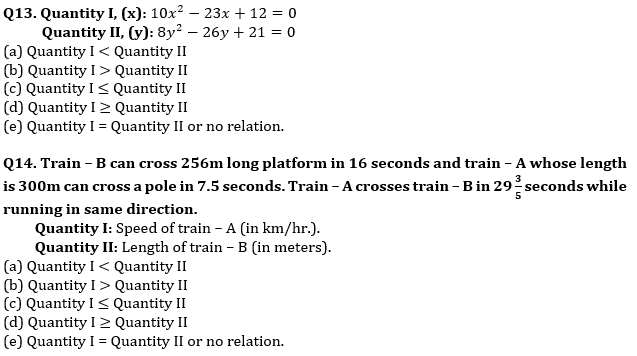Q15. Bag – A has 4 green and 6 blue balls and bag – B has 8 red balls, 5 green balls and 2 blue balls. A bag is picked randomly, and a ball is picked randomly from it.
Quantity I: Probability of ball being green ball.
Quantity II: Probability of ball being red ball.
(a) Quantity I < Quantity II
(b) Quantity I > Quantity II
(c) Quantity I ≤ Quantity II
(d) Quantity I ≥ Quantity II
(e) Quantity I = Quantity II or no relation.

Solutions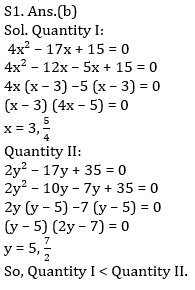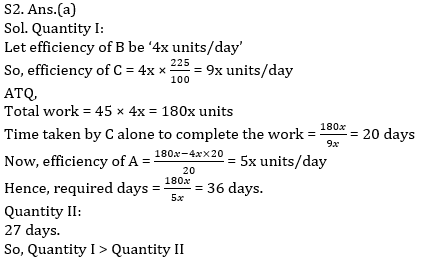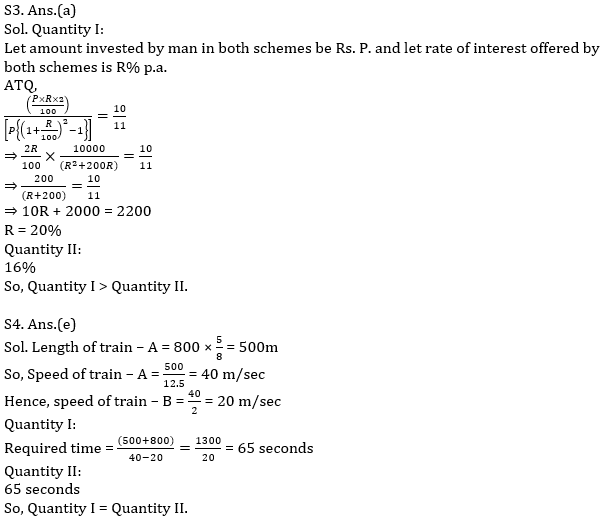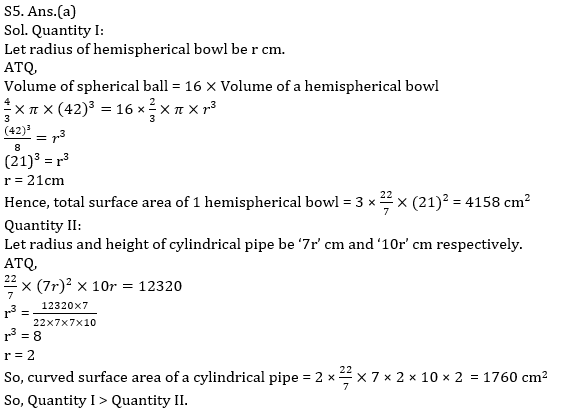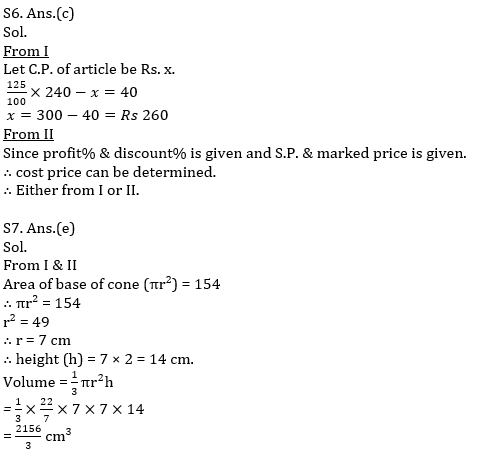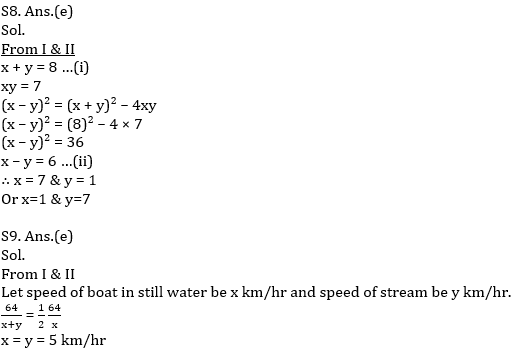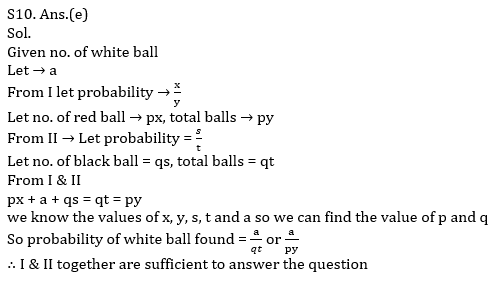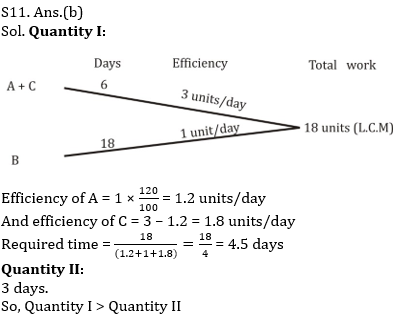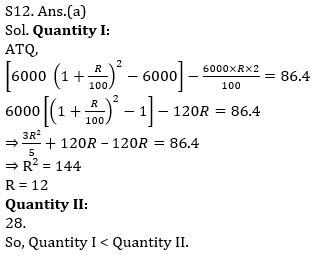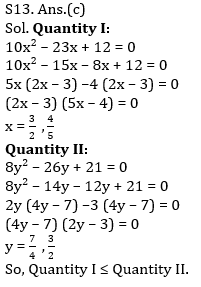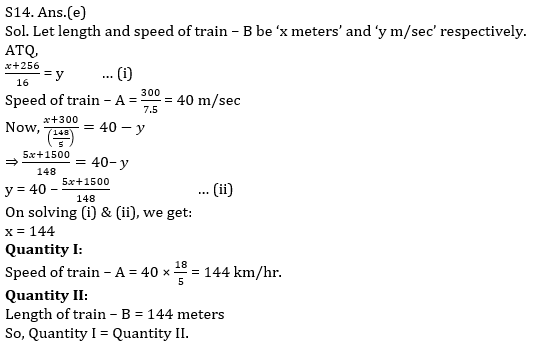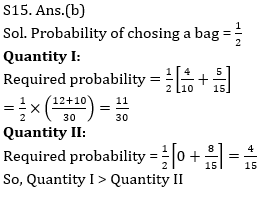×

Thank You, Your details have been submitted we will get back to you.Join India's largest learning destination

What You Will get ?

•Job Alerts
•Daily Quizzes
•Subject-Wise Quizzes
•Current Affairs
•Previous year question papers
•Doubt Solving session

ORJoin India's largest learning destination

What You Will get ?

•Job Alerts
•Daily Quizzes
•Subject-Wise Quizzes
•Current Affairs
•Previous year question papers
•Doubt Solving session

ORJoin India's largest learning destination

What You Will get ?

•Job Alerts
•Daily Quizzes
•Subject-Wise Quizzes
•Current Affairs
•Previous year question papers
•Doubt Solving session

Enter the email address associated with your account, and we'll email you an OTP to verify it's you.Join India's largest learning destination

What You Will get ?

•Job Alerts
•Daily Quizzes
•Subject-Wise Quizzes
•Current Affairs
•Previous year question papers
•Doubt Solving session

Enter OTP

Please enter the OTP sent to
/6

Did not recive OTP?

Resend in 60sJoin India's largest learning destination

What You Will get ?

•Job Alerts
•Daily Quizzes
•Subject-Wise Quizzes
•Current Affairs
•Previous year question papers
•Doubt Solving sessionJoin India's largest learning destination

What You Will get ?

•Job Alerts
•Daily Quizzes
•Subject-Wise Quizzes
•Current Affairs
•Previous year question papers
•Doubt Solving session

Almost there

+91Join India's largest learning destination

What You Will get ?

•Job Alerts
•Daily Quizzes
•Subject-Wise Quizzes
•Current Affairs
•Previous year question papers
•Doubt Solving session

Enter OTP

Please enter the OTP sent to Edit Number

Did not recive OTP?

Resend 60

By skipping this step you will not recieve any free content avalaible on adda247, also you will miss onto notification and job alerts

Are you sure you want to skip this step?

By skipping this step you will not recieve any free content avalaible on adda247, also you will miss onto notification and job alerts

Are you sure you want to skip this step?